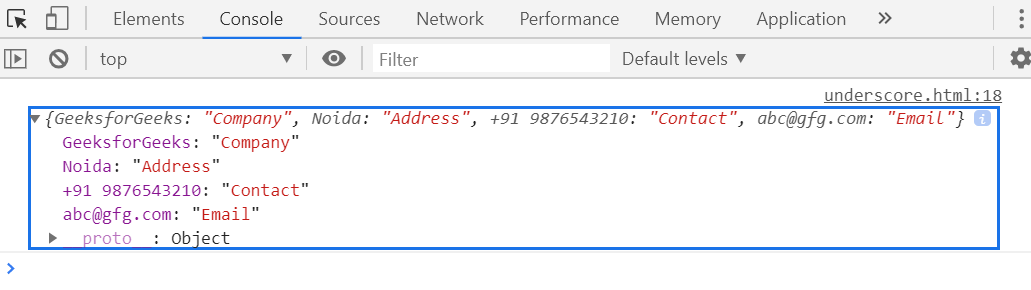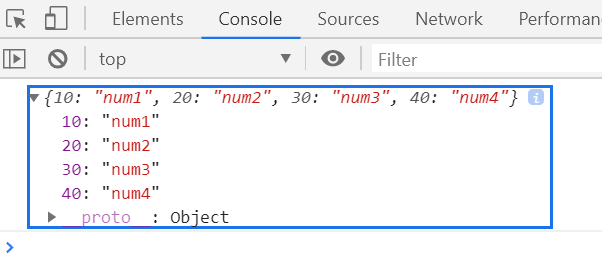Open in App
Not now

# Underscore.js _.invert() Function

• Last Updated : 25 Nov, 2021

The _.invert() function is used to return the copy of an object where the object key is converted into value and object value is converted into the key. It means the [key, value] of the object is reverse.

Syntax:

`_.invert( object )`

Parameters: This function accepts single parameter as mentioned above and described below:

• object: It contains the object element that holds the elements of key and value pair.

Return Value: It returns the [key, value] of an object in reverse [value, key] order.

Example 1:

 ```<``html``>`` ` `<``head``>``    ``<``script` `type``=``"text/javascript"` `            ``src``=``"https://cdnjs.cloudflare.com/ajax/libs/underscore.js/1.9.1/underscore-min.js"``>``    `````` ` `<``body``>``    ``<``script` `type``=``"text/javascript"``>`` ` `        ``var obj = {``            ``Company: "GeeksforGeeks",``            ``Address: "Noida",``            ``Contact: "+91 9876543210",``            ``Email: "abc@gfg.com"``        ``}``        ``console.log(_.invert(obj));``    `````` ` ``

Output:Example 2:

 ```<``html``>`` ` `<``head``>``    ``<``script` `type``=``"text/javascript"` `            ``src``=``"https://cdnjs.cloudflare.com/ajax/libs/underscore.js/1.9.1/underscore-min.js"``>``    `````` ` `<``body``>``    ``<``script` `type``=``"text/javascript"``>`` ` `        ``var inv = _.invert({``            ``num1: 10,``            ``num2: 20,``            ``num3: 30,``            ``num4: 40``        ``});`` ` `        ``console.log(inv);``    `````` ` ``

Output:My Personal Notes arrow_drop_up